Most Affordable JEE | NEET | 8,9,10 Preparation by Kota's Top IITian Doctor Faculties

# Important Questions of Organic Chemistry Class 11-With Solutions

Class 11`

Get General Organic Chemistry important questions for Class 11 exams. View the Important Question bank and notes for Class 11 & 12 Chemistry. These important questions will play significant role in clearing concepts of Organic Chemistry of class 11 which is base for competitive exams. This question bank is designed keeping NCERT in mind and the questions are updated with respect to upcoming Board exams. You will get here all the important questions for class 11 chemistry chapters. Learn the concepts of Organic Chemistry of Class 11 with the important questions and answers and prepare well for the exams.

Click Here for Detailed Chapter-wise Notes of Chemistry for Class 11th, JEE & NEET.  You can access free study material for all three subjects Physics, Chemistry and Mathematics. Click Here for Detailed Notes of any chapter.  eSaral provides you complete edge to prepare for Board and Competitive Exams like JEE, NEET, BITSAT, etc. We have transformed classroom in such a way that a student can study anytime anywhere. With the help of AI we have made the learning Personalized, adaptive and accessible for each and every one. Visit eSaral Website to download or view free study material for JEE & NEET. Also get to know about the strategies to Crack Exam in limited time period. Very Short Answer (1 Mark)

Q. Give the IUPAC names of the following compounds :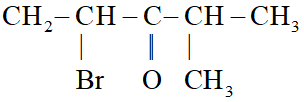Ans. 2-bromo- 4-methyl pentan- 3-one
Q. Give the IUPAC name of the alkane having the lowest molecular mass that contain a quaternary carbon.
Ans.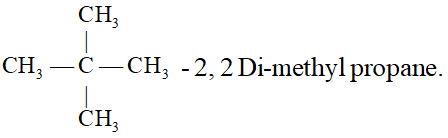Q. Write the structural formula of (i) sec-butyl and (ii) isobutyl groups.
Ans.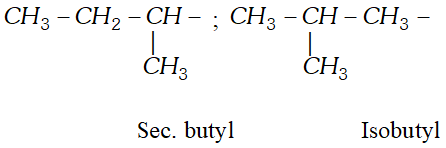Q. Lassaigne’s test is not shown by diazonium salts, though they contain nitrogen. Why ?
Ans. Diazonium salts $\left(C_{6} H_{5} N_{2}^{+} X^{-}\right)$ readily lose $N_{2}$ on heating before reacting with fused sodium metal. Therefore, these do not give positive Lassaigne's test for nitrogen.
Q. Suggest a suitable technique of separating naphthalene from kerosene present in a mixture.
Ans. By differential extraction.
Q. How will you separate a mixture of o-nitro-phenol and p-nitrophenol ?
Ans. A mixture of o-nitrophenol and p-nitrophenol can be separated by steam distillation. o-nitrophenol being less volatile distils over along with water while p-nitrophenol being non-volatile remains in the flask.
Q. Will $C C l_{4}$ give white precipitate of $A g C l$ on heating with silver nitrate? Give reason for your answer.
Ans. The precipitate of $A g C l$ will not be formed because $C C l_{4}$ is covalent compound and does not ionise to give $C l^{-}$ ions to react with $A g N O_{3}$. $C C l_{4}+A g N O_{3} \longrightarrow$ No reaction
Q. Which is expected to be more stable, $\mathrm{O}_{2} N \mathrm{CH}_{2} \mathrm{CHO}$ or $\mathrm{CH}_{3} \mathrm{CH}_{2} \mathrm{O}^{-}$ and why?
Ans. $\mathrm{O}_{2} \mathrm{N} \mathrm{CH}_{2} \mathrm{CHO}$ is expected to be more stable because each atom has complete its octet and has no charge.
Q. How many $\sigma$ and $\pi$ bonds are present in each of the following molecules? (i) $\quad \mathrm{CH}_{3}-\mathrm{CH}=\mathrm{CH}-\mathrm{C} \equiv \mathrm{CH}$ (ii) $\quad \mathrm{HC} \equiv \mathrm{C}-\mathrm{CH}_{2}-\mathrm{CH}=\mathrm{CH}_{2}$
Ans. (i) $\quad \sigma_{C-C}=4, \sigma_{C-H}=6, \pi_{C=C}=3$ (ii) $\quad \sigma_{\mathrm{C}-\mathrm{C}}=4, \sigma_{\mathrm{C}-\mathrm{H}}=6, \pi_{\mathrm{C}=\mathrm{C}}=3$
Q. Which of the following pairs of structures do not constitute resonance structures :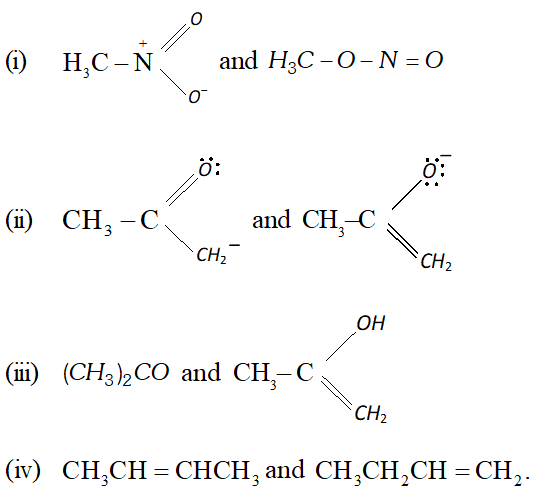Ans. Pair of structures shown in (i) and (iv) do not constitute resonance structures.
Q. Classify the following molecules/ions as nucleophiles or electrophiles: $H \ddot{S}^{-}, B F_{3}, C H_{3} C H_{2} O^{-}$ $\left(\mathrm{CH}_{3}\right)_{3} \mathrm{N}, \mathrm{Cl}^{+}, \mathrm{CH}_{3} \mathrm{C}=\mathrm{O}, \mathrm{H}_{2} \mathrm{N}:, \mathrm{NO}_{2}^{+-}$
Ans. Electrophiles : $B F_{3} . C l^{+}, C H_{3}^{+} C=O, N O_{2}^{+}$ Nucleophiles : $H S, C H_{3} C H_{2} O^{-},\left(C H_{3}\right)_{3} \ddot{N}, \dot{H}_{2} \bar{N}$:
Q. In $s p^{3}, s p^{2}$ and sp hybrid orbitals which hybrid orbitals show the electronegativity?
Ans. $s p^{3}$ hybrid orbitals.
Q. What type of hybridization is shown by second carbon atom.
Ans. sp hybridization.
Q. Explain why $\left(\mathrm{CH}_{3}\right)_{3} \mathrm{C}^{+}$ is more stable than $C H_{3}^{+} C H_{2}$ and $^{+} C H_{3}$ is the least stable cation.
Ans.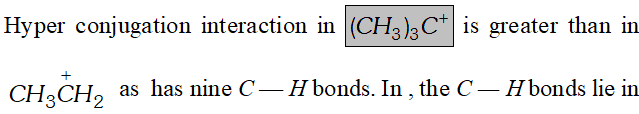the nodal plane of the vacant 2p orbitals and hence cannot overlap with it. Thus lacks hyperconjugative stability.
Q. State bond length and strength of $s p, s p^{2}$ and $s p^{3}$ hybrid orbitals.
Ans. The sp hybrid orbital contains more $s$ character and hence it is closer to its nucleus and forms shorter and stronger bonds than the $s p^{3}$ hybrid orbital. The $s p^{2}$ hybrid orbital is intermediate between $s p$ and hybrid orbitals, hence the length and enthalpy of the bonds it forms is also intermediate between them.
Q. On the basis of type of hybridization, predict the shape of the following molecules : (i) $\quad H_{2} C=O \quad$ (ii) $C H_{3} F \quad$ (iii) $\mathrm{HC} \equiv \mathrm{N}$
Ans. (i) $s p^{2}$ hybridised carbon and therefore, shape is trigonal planar. (ii) $s p^{3}$ hybridised carbon and shape is tetrahedral. (iii) $s p$ hybridised carbon and shape is linear.
Short Answer (2 or 3 Marks)
Q. Write IUPAC nomenclature of the following :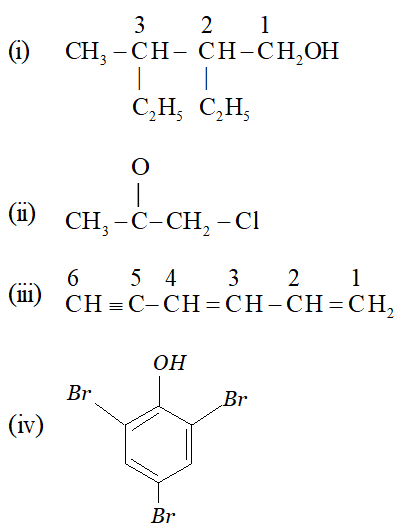Ans. (i) 2-ethyl 3-methyl pentan-1-ol (ii) 1-chloro propan-2-one (iii) Hexa 1, 3-diene-5-yne (iv) 2, 4, 6 – tri bromo phenol
Q. Give IUPAC name for the following :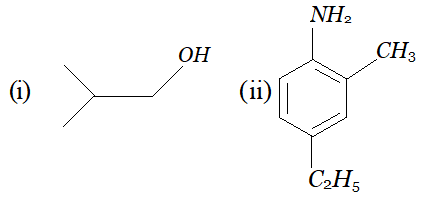Ans. (i) 2 methyl propan-1-ol (ii) 4-ethyl-2-methyl aniline
Q. Give IUPAC name of the following organic molecules :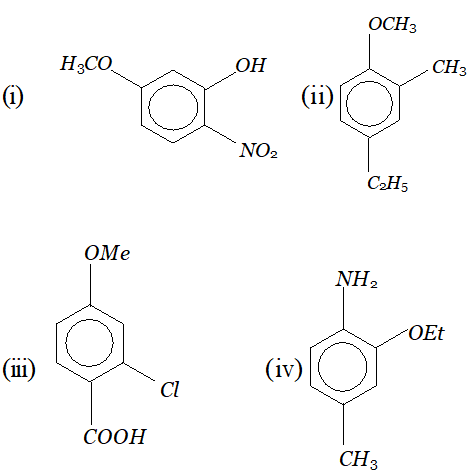Ans. (i) 5-methoxy-2-nitrophenol (ii) 4-ethyl-2-methyl anisol (iii) 2-chloro-4-methoxy benzoic acid (iv) 2-ethoxy-4-methyl aniline
Q. Write the IUPAC names of :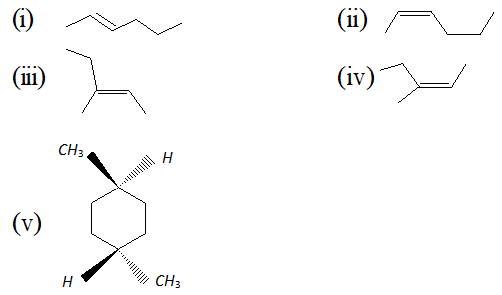Ans. (i) Trans-2-hexene (ii) Cis-2-hexene (iii) (E)-3-methyl-2-pentene (iv) (Z)-3-methyl-2-pentene (v) Trans-1, 4-dimethyl cyclo hexane.
Q. Give the IUPAC name of the following compounds :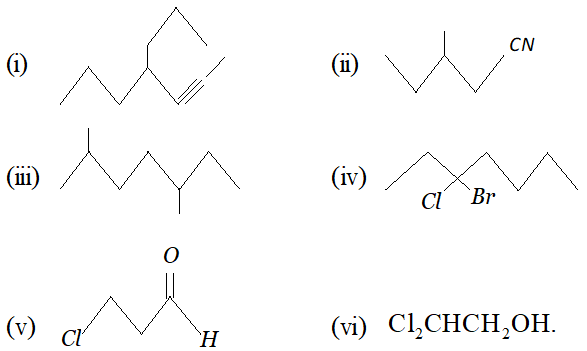Ans.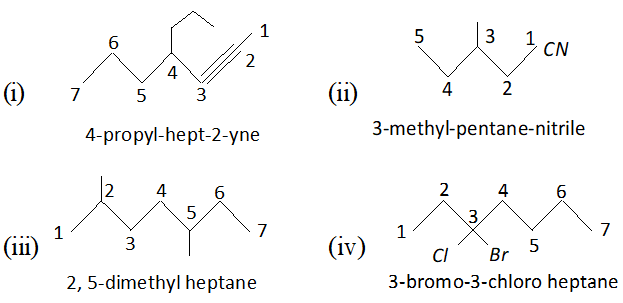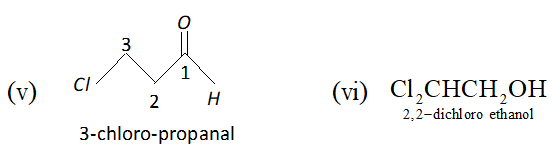Q. Which of the following represents the correct IUPAC name for the compounds concerned : (i) 2, 2–dimethylpentane or 2–dimethylpentane. (ii) 2, 3–dimethyl pentane or 3, 4–dimethyl pentane. (iii) 2, 4, 7–trimethyloctane or 2, 5, 7–trimethyloctane, (iv) 2 –chloro–4–methylpentane or 4–chloro 2–methylpentane, (v) But–3–yn-1-ol or But–4–ol–1–yne. [NCERT]

Ans. (i) 2, 2-dimethyl pentane (ii) 2, 3-dimethyl pentane (iii) 2, 4, 7-trimethyl octane (iv) 2-chloro-4-methyl pentane (v) But-3-yne-1-ol
Q. Write IUPAC name of the following :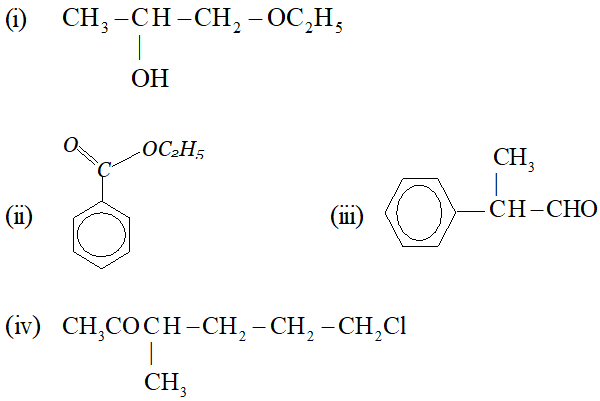Ans. (i) 1-ethoxy propan-2-ol (ii) Ethyl benzoate (iii) 2-phenyl propanal (iv) 6-chloro-3-methyl-hexan-2-one
Q. Write bondline formulae for : tert–Butylcyclopentane, Isopropyl alcohol, 2,3-Dimethylbutanal, Heptan-4-one, Cyclohexanone.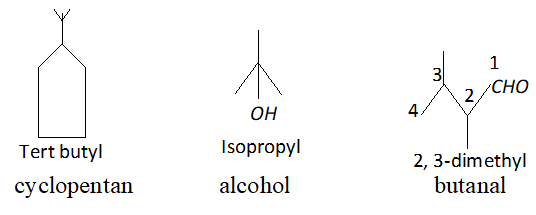Ans.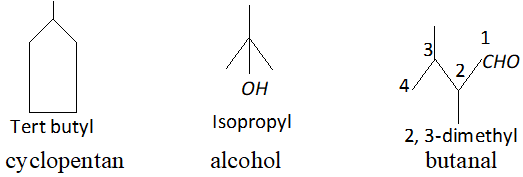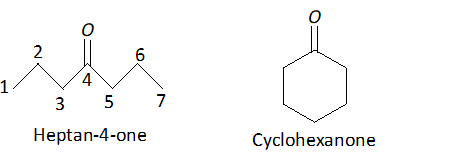Q. Draw formulae for the first five members of each homologous series beginning with the following compounds. (i) $\mathrm{HCOOH},$ (ii) $\mathrm{CH}_{3} \mathrm{COCH}_{3},\left(\text { iii) } \mathrm{CH}_{2}=\mathrm{CH}_{2}\right.$
Ans.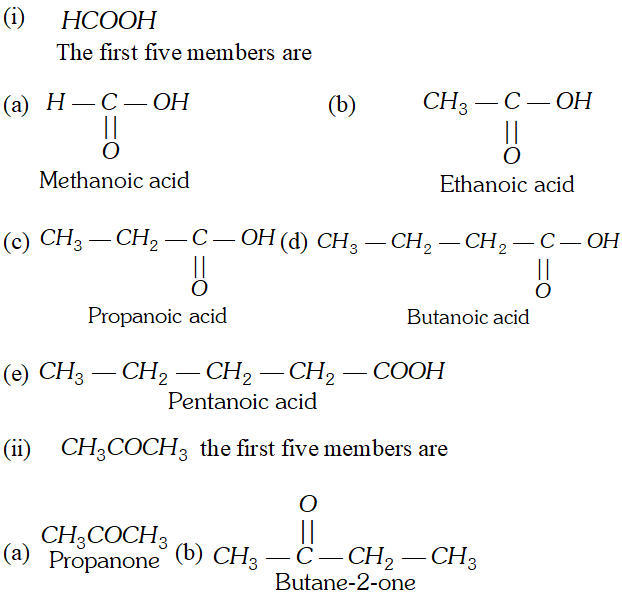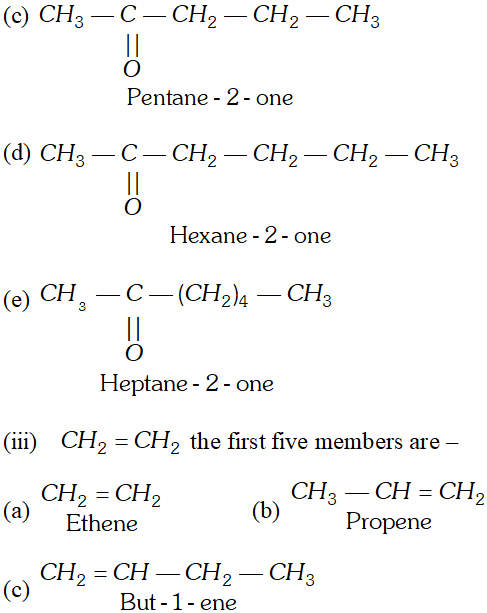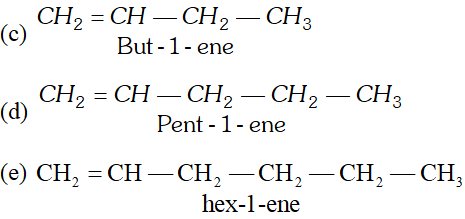Q. Identify the functional groups in the following compounds: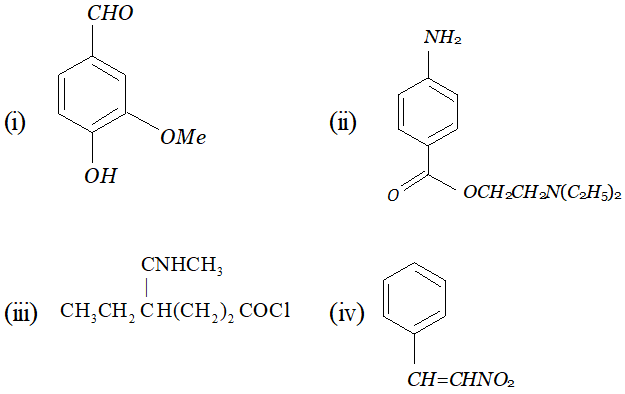Ans.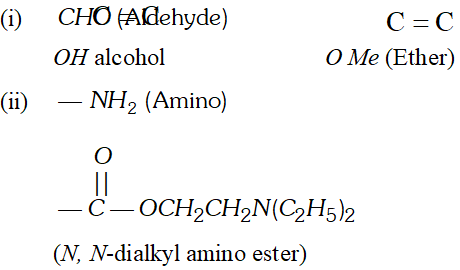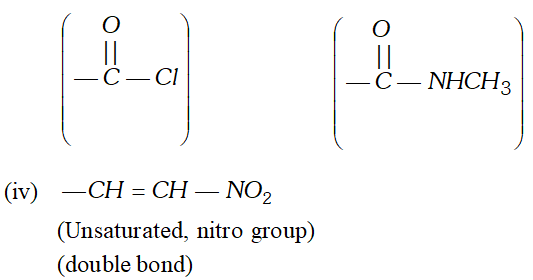(iii) N-Substituted amide and acid chloride
Q. For the following bond cleavages, use curved-arrows to show the electron flow and classify each as homolysis or heterolysis. Identify reactive intermediate products as free radical, carbocation and carbanion.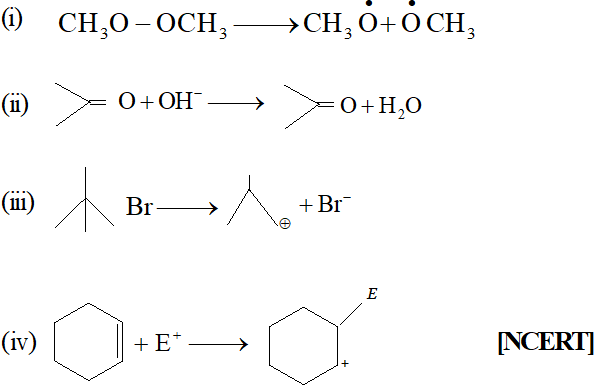[NCERT]
Ans. (i) Homolysis, Free radicals are formed.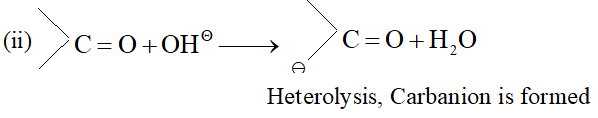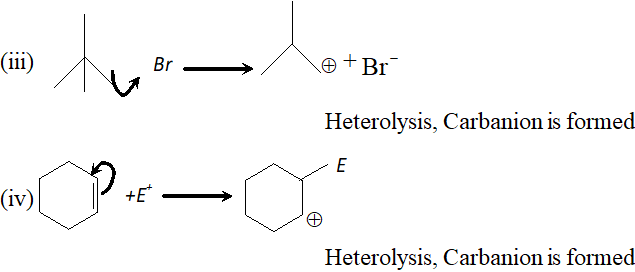Q. Explain the order of stability of primary, secondary and tertiary carbonium ions.
Ans. $3^{\circ}>2^{\circ}>1^{\circ}$ is order of stability of carbocation. 3 " carbocation are most stable due to maximum positive inductive effect of three alkyl groups. $2^{\circ}$ carbocation is morestable than $1^{\circ}$ carbocation due togreater inductive effect of two alkyl groups as compared to $1^{\circ} C$ carbocation in which there is one alkyl group.
Q. 0.378 g of an organic acid gave on combustion 0.264 g of carbon dioxide and 0.162 g of water vapours. Calculate the percentage of C and H. [NCERT]

Ans. Mass of organic compound $=0.378 \mathrm{g}$ Mass of $\mathrm{CO}_{2}$ formed $=0.264 \mathrm{g}$ Mass of $\mathrm{H}_{2} \mathrm{O}$ formed $=0.162 \mathrm{g}$ (i) Percentage of carbon $44 \mathrm{g}$ of contains carbon $0.264 \mathrm{g}$ of contains carbon $=12 \mathrm{g}$ $=\frac{12}{44} \times 0.264=0.072 \mathrm{g}$ Percentage of carbon $=\frac{0.072}{0.378} \times 100=19.04 \%$ $18 g$ of contains hydrogen $=2 g$ $0.162 g$ of contains hydrogen $=\frac{2}{18} \times 0.162=0.018 \mathrm{g}$ Percentage of hydrogen $=\frac{0.018}{0.378} \times 100=4.76 \%$ (ii) Percentage of hydrogen
Q. During nitrogen estimation of an organic compound by Kjeldahl's method, the ammonia evolved by $0.5 g$ of the compound neutralised $10 \mathrm{mL}$ of $1 \mathrm{M} \mathrm{H}_{2} \mathrm{SO}_{4} .$ Calculate the percentage of nitrogen in the compound.
Ans. $1 \mathrm{M}$ of $10 \mathrm{mL} \mathrm{H}_{2} \mathrm{SO}_{4} \equiv 1 \mathrm{M}$ of $20 \mathrm{mL}$ of $\mathrm{NH}_{3}$ $1000 \mathrm{mL}$ of $1 \mathrm{M}$ ammonia contains $=14 \mathrm{g}$ nitrogen $20 \mathrm{mL}$ of $1 \mathrm{M}$ ammonia contain $=\frac{14 \times 20}{1000} \mathrm{g}$ nitrogen $\therefore$ Percentage of nitrogen $=\frac{14 \times 20}{1000 \times 0.5} \times 100=56.0 \%$
Q. (i) In sulphur estimation, 0.157 g of organic compound gave $0.4813 \mathrm{g}$ of $\mathrm{BaSO}_{4} .$ What is the percentage of sulphur in organic compound? (ii) $0.092 g$ of organic compound on heating in carius tube and subsequence ignition gave $0.111 g$ of $M g_{2} P_{2} O_{7}$. Calculate the percentage of phosphorus in organic compound.
Ans. (i) Mass of $B a S O_{4}=0.4813 \mathrm{g}$ Mass of organic compound $=0.157 \mathrm{g}$ $\% \mathrm{S}=\frac{32 \times W_{B a S O_{4}} \times 100}{233 \times W_{\mathrm{Substance}}}=\frac{32 \times 0.4813 \times 100}{233 \times 0.157}=42.10$ (ii) Mass of organic compound $=0.092 \mathrm{g}$ Mass of $\mathrm{Mg}_{2} \mathrm{P}_{2} \mathrm{O}_{7}=0.111 \mathrm{g}$ $\mathrm{g}_{\mathrm{bof}} \mathrm{P}=\frac{62 \times \mathrm{W}_{\mathrm{Mg}_{2} \mathrm{P}_{2} \mathrm{O}_{7}}}{222 \times \mathrm{W}_{\mathrm{Substance}}} \times 10 \mathrm{C}=\frac{62 \times 0.111 \times 100}{222 \times 0.092}=33690024 Q. (i) A mixture contains benzoic acid and nitrobenzene. How can this mixture be separated into its constituents by the technique of extraction using an appropriate chemical reagent? (ii) Write the formula of iron (III) hexa cyanoferrate (II). [NCERT] Ans. (i) The mixture is shaken with a dilute solution of$\mathrm{NaHCO}_{3}$and extracted with ether or chloroform when nitrobenzene goes into the organic layer. Distillation of the solvent gives nitrobenzene. The filtrate is acidified with dil. HCl when benzoic acid gets precipitated. The solution is cooled and benzoic acid is obtained by filtration (ii)$\quad F e_{4}\left[F e(C N)_{6}\right]$Q. Give hybridization state of each carbon in following compounds :$\mathrm{CH}_{2}=\mathrm{C}=\mathrm{O}, \mathrm{CH}_{3}-\mathrm{CH}=\mathrm{CH}_{2},\left(\mathrm{CH}_{3}\right)_{2} \mathrm{CO}, \mathrm{CH}_{2}=\mathrm{CHCN},\mathrm{CH}_{3} \mathrm{CH}_{2}^{-}, \mathrm{CH}_{3} \mathrm{CH}_{2}^{+}, \mathrm{CH}_{3} \mathrm{CH}_{2}$Ans.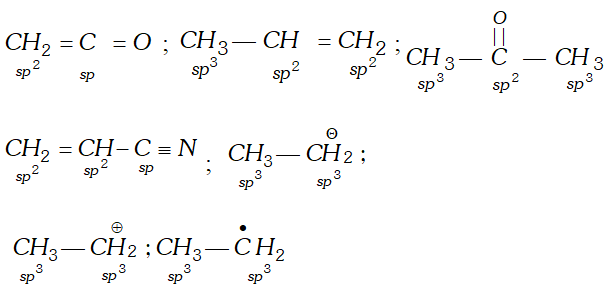Q. (i) Giving justification categorise the following species as nucleophile or electrophile :$\begin{array}{ll}{\text { (a) } B F_{3}} & {\text { (b) } C_{2} H_{5} O^{-}}\end{array}$(ii) Write the IUPAC names of the following compounds :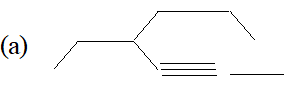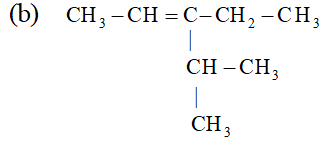Ans. (i)$\quad$(a)$B F_{3}$is electrophile because it is electron deficient, i.e. octet of boron is not complete. (b)$C_{2} H_{5} O^{-}$is nucleophile because it is negatively charged.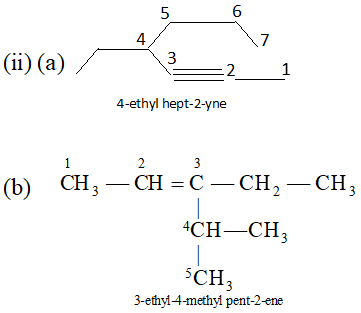Q. Indicate the$\sigma$and$\pi$-bonds in the following molecules: (i)$\quad C_{6} H_{6}$(ii)$\quad C_{6} H_{12}$(iii)$C H_{2} C l_{2}$(iv)$\quad \mathrm{CH}_{3} \mathrm{NO}_{2}$(v)$\quad H C O N H C H_{3}$[NCERT] Ans. (i)$\quad C_{6} H_{6}$is benzene and structural formulae is: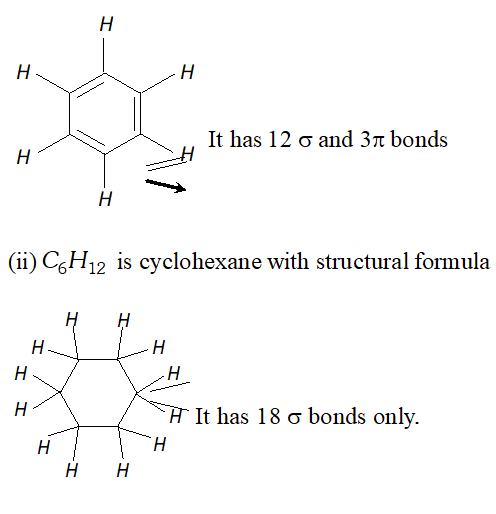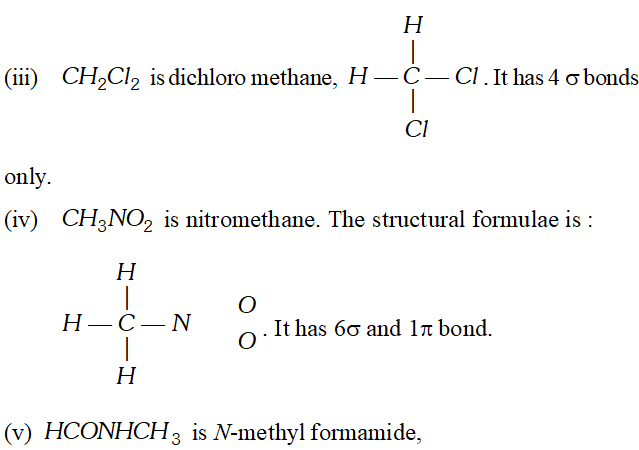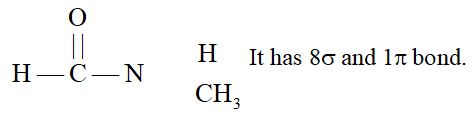Q. Draw the complete structures of bromomethane, bromoethane. 2–bromopropane and tert–butylbromide. Arrange them in order of decreasing steric hindrance. [NCERT] Ans.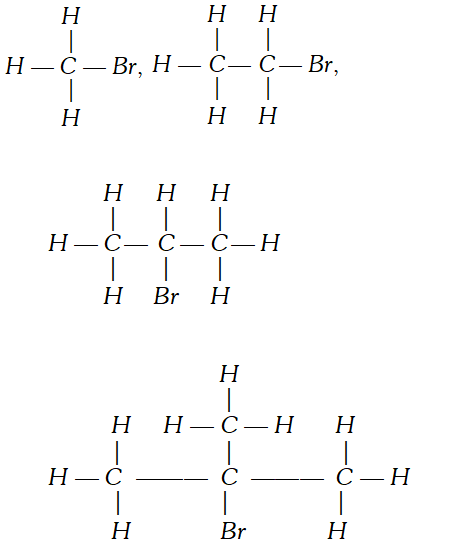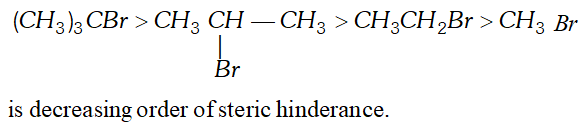Q. Draw the resonating structures for the following compounds. Show the electron shift using curved arrow notation. (i)$\quad C_{6} H_{5} O H$(ii)$\quad C_{6} H_{5} N O_{2}$(iii)$C_{6} H_{5} C^{\oplus} H_{2}$(iv)$\quad \mathrm{CH}_{3} \mathrm{CH}=\mathrm{CHCHO}$(v)$\quad \mathrm{CH}_{3} \mathrm{CH}=\mathrm{CH}-\mathrm{CH}_{2}^{\oplus}$(vi)$\quad C_{6} H_{5} \mathrm{CHO}$(vii)$C H_{2}=C H O C H_{3}$[NCERT] Ans.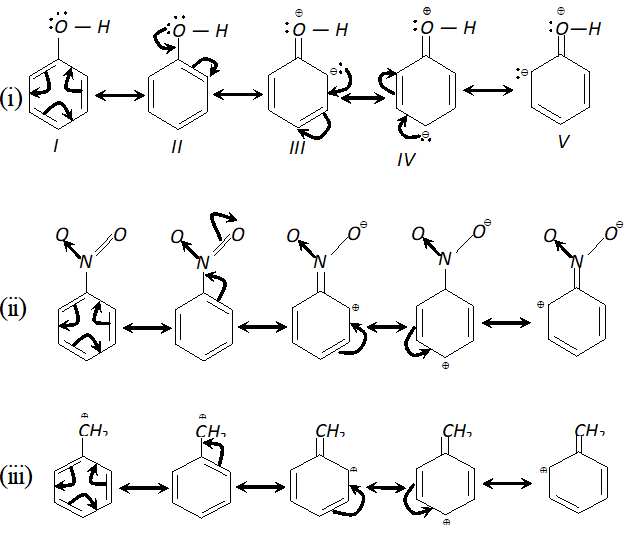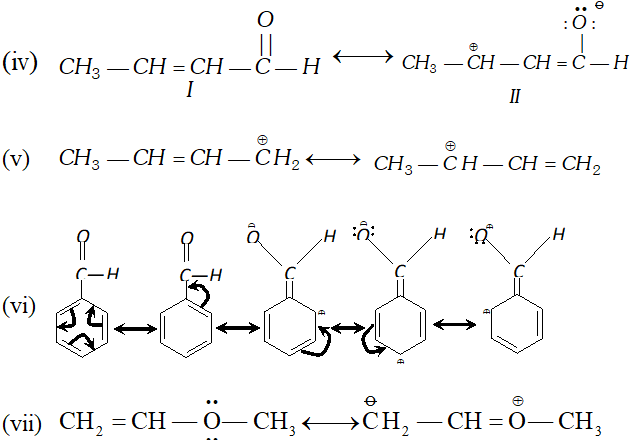Q. Explain why alkyl groups act as electron donors when attached to a$\pi-$system. [NCERT] Ans. Alkyl group has$s p^{3}$hybridization whereas$\pi$-bond atom is$s p^{2}$hybridized which is more electronegative therefore alkyl group acts as electron donors. Q. Classify the reagents shown in bold in the following equation as nucleophiles or electrophiles. Use curved-arrow notation to show the electron movement. (i)$\mathrm{CH}_{3} \mathrm{COOH}+\mathrm{HO}^{-} \longrightarrow \mathrm{CH}_{3} \mathrm{COO}^{-}+\mathrm{H}_{2} \mathrm{O}$(ii)$\mathrm{CH}_{3} \mathrm{COCH}_{3}+^{-} \mathrm{NC} \longrightarrow \mathrm{CH}_{3} \mathrm{C}(\mathrm{CN}) \mathrm{OHCH}_{3}$(üii)$\mathrm{C}_{6} \mathrm{H}_{6}+\mathrm{CH}_{3} \mathrm{C}^{+} \mathrm{O} \longrightarrow \mathrm{C}_{6} \mathrm{H}_{5} \mathrm{COCH}_{3}$[NCERT] Ans. (i)$\quad O H^{-}$is nucleophile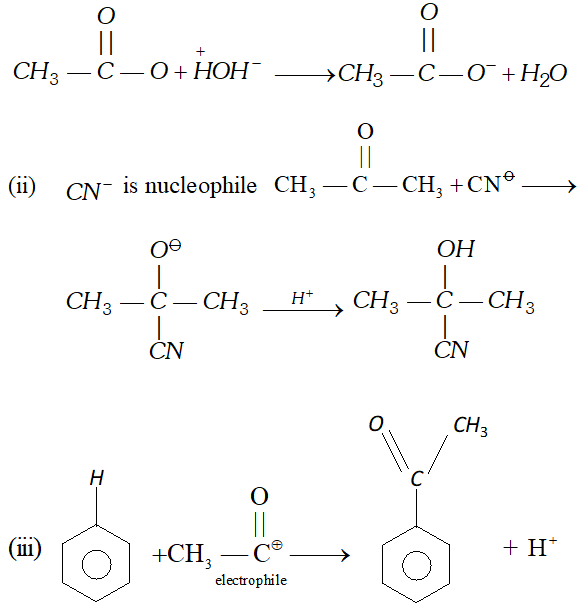Q. Classify the following reactions in one of the reaction type: (i)$\mathrm{CH}_{3} \mathrm{CH}_{2} \mathrm{Br}+\mathrm{HS}^{-} \longrightarrow \mathrm{CH}_{3} \mathrm{CH}_{2} \mathrm{SH}+\mathrm{Br}^{-}$(ii)$\left(\mathrm{CH}_{3}\right)_{2} \mathrm{C}=\mathrm{CH}_{2}+\mathrm{HCl} \longrightarrow\left(\mathrm{CH}_{3}\right)_{2} \mathrm{ClC}-\mathrm{CH}_{3}$(iii)$\left.\mathrm{(CH}_{3}\right)_{2} \mathrm{CH}-\mathrm{CH}_{2} \mathrm{CH}_{2} \mathrm{OH}+\mathrm{HBr} \rightarrow\left(\mathrm{CH}_{3}\right)_{2} \mathrm{CBr}_{2} \mathrm{CH}_{3}$(iv)$\mathrm{CH}_{3} \mathrm{CH}_{2} \mathrm{Br}+\mathrm{HO} \longrightarrow \mathrm{CH}_{2}=\mathrm{CH}_{2}$[NCERT] Ans. (i) Nucleophilic Substitution reaction (ii) Addition reaction (iii) Nucleophilic Substitution reaction (iv) Elimination reaction. Q. Explain with the help of examples that Geometrical isomerism is different from conformation. Ans. Conformation are obtained by rotation around$\sigma$-bond, e.g., eclipsed and staggered conformation of ethane.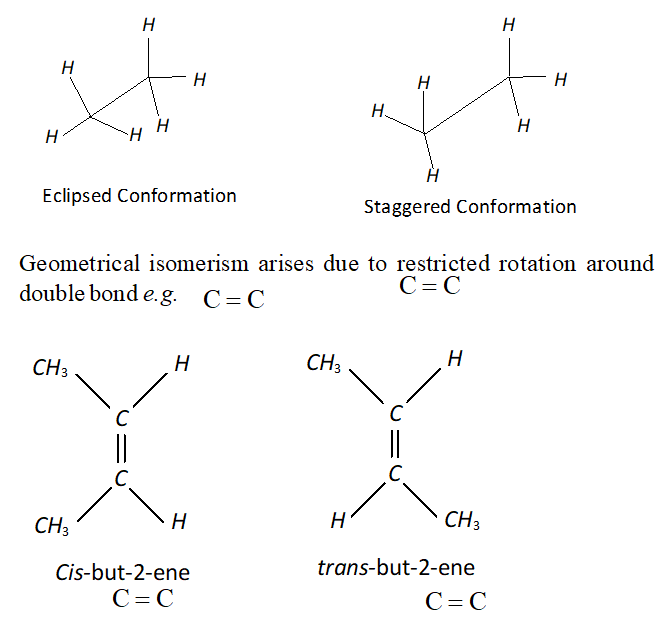#### India's Best Exam Preparation for Class 11th - Download Now Q. What is the relationship between the members of following pairs of structures ? Are they identical, structural or geometrical isomers, or resonance contributors ?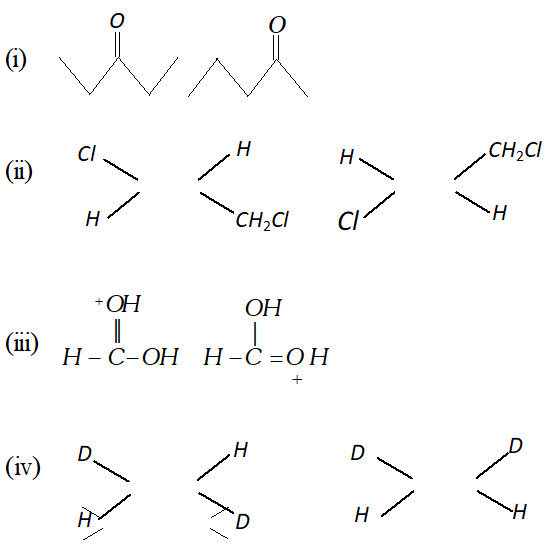Ans. (i) Structural Isomers. (ii) Geometrical Isomers. (iii) Resonating structures. (iv) Geometrical Isomers. Long Answer (5 Mark) Q. (i) Given the IUPAC name for the amine.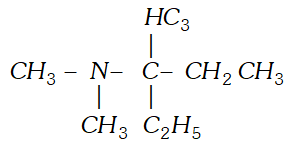(ii) Discuss the hybridization of carbon atoms in allene$\left(C_{3} H_{4}\right)$and show the$\pi$- orbital overlaps. Ans. (i)$3, N, N$-Trimethylpentan-$-3$-amine. (ii)$\quad$The structure of allene$\left(C_{3} H_{4}\right)$is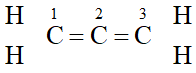The carbon atoms 1 and 3 are$s p^{2}$-hybridized since each one of them is joined by a double bond. In contrast, carbon atom 2 is -hybridized since it has two double bonds. Thus, the two$\pi-$bonds in allene like in acetylene are perpendicular to each other as shown below :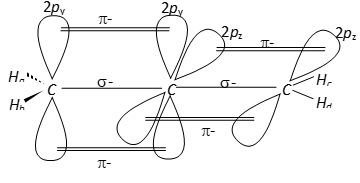Whereas$H_{c}$and$H_{d}$lie in the plane of the paper while$H_{a}$and$H_{b}$lie in a plane perpendicular to the plane of the paper. Q. Give IUPAC names of the following compounds :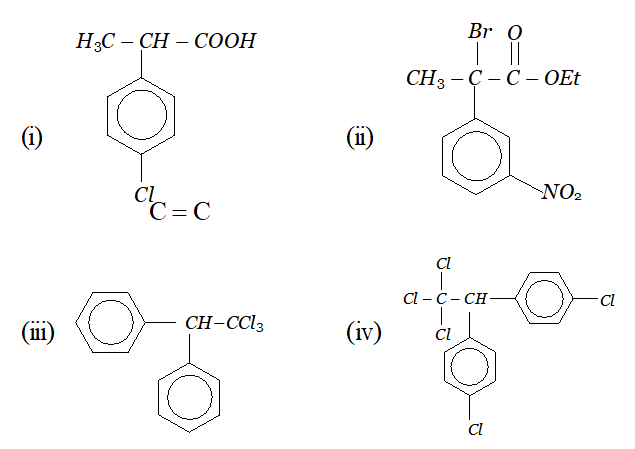Ans. In all the questions given above the ring phenyl group. In case the phenyl group is further substituted, the carbon atoms of the ring are separately numbered starting from the carbon atom of the ring directly attached to the parent chain in such a way that substituent on ring gets lowest possible position. According the IUPAC name are : (i) 2-(4-chlorophenyl) propanoic acid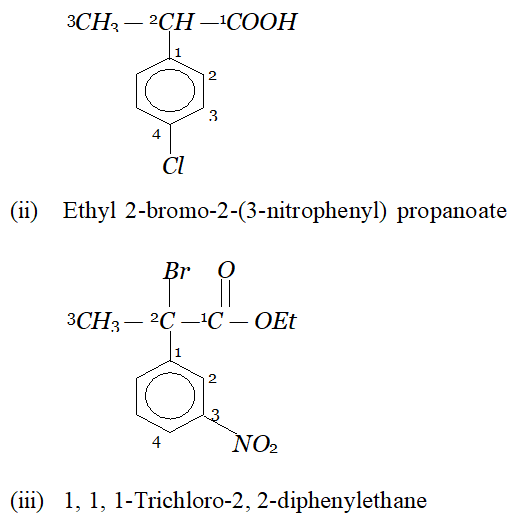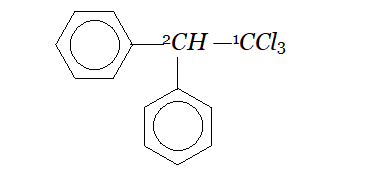(iv) If the compound contains more than one similar complex radicals/substituents, then the numerical prefixes such as di, tri, tetra, etc. are replaced by bis,tris, tetrakis, etc. respectively. Hence, the IUPAC name is : 1, 1, 1-Trichloro-2, 2-bis-(chlorophenyl) ethane.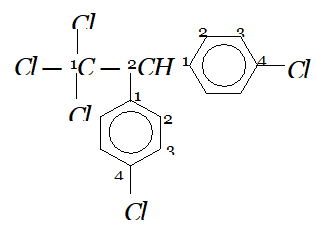Q. (i) A mixture contains two components A and B. The solubilities of A and B in water near its boiling point are 10 grams per 100 ml and 2 g per 100 ml respectively. How will you separate A and B from this mixture? (ii) Explain why an organic liquid vapourizes at a temperature below its boiling point in steam distillation? (iii) Which technique can be used to separate naphthalene from kerosene oil present in its mixture. [NCERT] Ans. (i) A and B from the mixture can be separated by using Fractional crystallization. When the saturated hot solution of this mixture is allowed to cool, the less soluble component B crystallizes out first leaving the more soluble component A in the mother liquor. (ii) A liquid boils when its vapour pressure becomes equal to the atmospheric pressure. During steam distillation, the mixture boils when sum of the vapour pressure of water and organic liquid becomes equal to the atmospheric pressure. Since the vapour pressure of water is appreciably higher than that of organic liquid, therefore, the organic liquid will vapourize at a temperature much lower than its normal boiling point. (iii) Since naphthalene and kerosene have large difference in their boiling points. Hence they can be separated by simple distillation. Q. (i) The$R_{f}$value of A and B in a mixture determined by TLC in a solvent mixture are 0.65 and 0.42 respectively. If the mixture is separated by column, chromatography using the same solvent mixture as a mobile phase, which of the two components, A or B, will elute first ? Explain. (ii) A mixture contains 71 percent calcium sulphate and 29 percent camphor. Name a suitable technique of separation of the components of this mixture. (iii) Without using column chromatography, how will you separate a mixture of camphor and benzoic acid? [NCERT] Ans. (i) Since the$R_{f}$value of A is 0.65, therefore, it is less strongly adsorbed as compared to compound B with value of 0.42. Therefore, on extraction of the column, A will elute first. (ii) Sublimation process can be used for the separation of camphor and calcium sulphate. Camphor sublimes where as is non-volatile. (iii) Sublimation cannot be used since both camphor and benzoic acid sublime on heating. Therefore, a chemical method using solution is used when benzoic acid dissolves leaving camphor behind. The filtrate is cooled and then acidified with dil. HCl to get benzoic acid. Q. What are reactive intermediates ? How are they generated by bond fission? [NCERT] Ans. Generally, the organic reactions complete through the involvement of certain chemical species which are short lived ($10^{-6}$seconds to a few seconds) and highly reactive. The short lived and highly reactive species are called reactive intermediates such as carbocations, carboanions, free radicals, carbenes and nitrenes. Heterolytic fission of covalent bond results in carbocations and carboanions. Free radicals are formed by homolytic fission. Carbenes are neutral carbon species in which carbon atom is bonded to two monovalent atoms or groups and also contains one non-bonding pair of electrons. They are produced in photolysis (irradiation with UV light or thermolysis or pyrolysis (action of heat) on diazoalkanes or ketones.)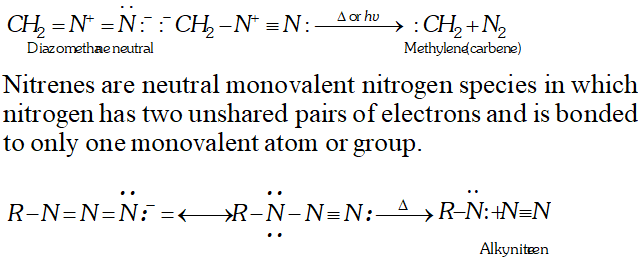Q. What is the relationship between the members of following pairs of structures ? Are they identical, structural or geometrical isomers, or resonance contributors ?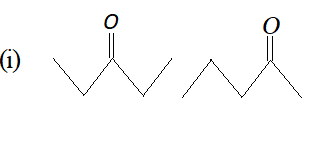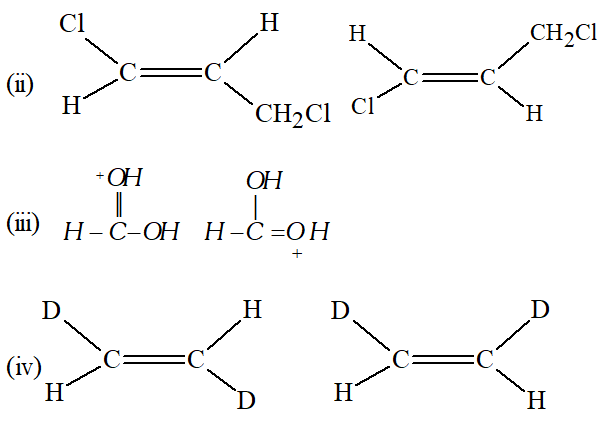Ans. (i) Structural Isomers. (ii) Geometrical Isomers. (iii) Resonating structures. (iv) Geometrical Isomers. Q. What are the different types of structural isomerism ? Describe them with suitable examples. Ans. Structural isomerism is of following types : (i) Chain isomerism : “When two or more compounds have same molecular formula but different carbon skeleton are said to have chain isomerism.” For example,$\mathrm{C}_{5} \mathrm{H}_{12}$shows the following three isomers: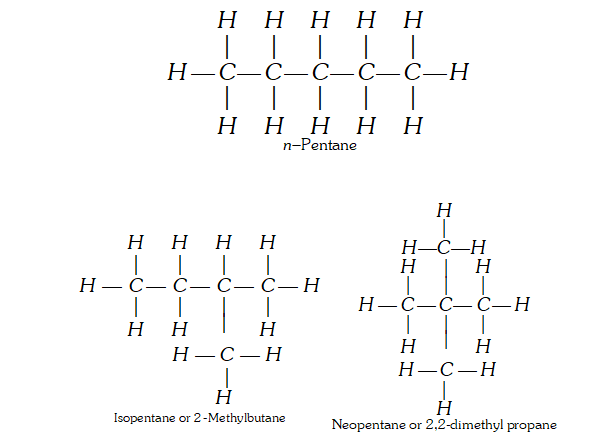(ii) Position Isomerism : ‘When two or more compounds have same molecular formula but different position of substituent atoms or groups on the carbon skeleton, are said to have position isomerism.’ For example,$C_{3} H_{8} O$shows the following two position isomers of alcohol :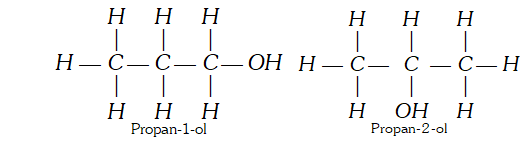(iii) Functional group isomerism : ‘When two or more compounds have same molecular formula but different functional groups, are said to have functional group isomerism.’ For example,$C_{4} H_{10} O\$ represents the following two compounds.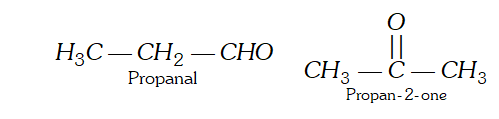Q. Why do alkenes and cycloalkanes show cis-trans isomerism? Or What is the origin of geometrical isomerism in alkenes?
Ans. Presence of carbon-carbon double bond, in a molecule is the cause of geometrical isomerism. This is because when a carbon-carbon double bond is present, the molecule cannot rotate freely about the double bond. It is called hindered or restricted rotation of the molecule about the double bond. During the formation of double bond, if two similar atoms or groups are present on the same side of the plane of the double bond it is called a cis isomer and if present on the opposite sides, it is called a trans isomer. Because of restriction in free rotation one form cannot change to the other. Therefore presence of C = C bond is the origin of geometrical isomerism . This restriction in rotation may be present in rigid of cyclic molecules. Therefore cyclic compounds also shows geometrical isomerism.

Tanisha
Aug. 9, 2023, 6:35 a.m.
These questions are very nice
Tanisha
Aug. 9, 2023, 6:35 a.m.
These questions are very nice
Sumit
Aug. 2, 2023, 6:35 a.m.
Hindi medium ke liye bhi note Bane chye na
Om garg
June 19, 2023, 6:35 a.m.
Fully satisfied with your questions you help.me a lot in doing my practice for IIT Thankyou so much♥️
suman tharu
April 23, 2023, 6:35 a.m.
All Important question of IUPAC name class 11 chemistry
Sugarxx
March 19, 2023, 6:35 a.m.
The questions are amazing !!! I enjoyed it 💝💫
Bhavishya
Feb. 18, 2023, 11:24 a.m.
These questions are too good. I like it 👌
Bhavishya
Feb. 18, 2023, 11:24 a.m.
These questions are too good I like it 👌
Ritu
Nov. 24, 2022, 8:53 p.m.
Thank you for this question answers. This questiobs is very useful for me .
Jaspreet Kaler
Nov. 22, 2022, 10:05 p.m.
Thanks for the questions if really helped a lot 😊😊
Deepti
Aug. 7, 2023, 6:35 a.m.
Thank you a lot
Apoorva Manjunath Machenahalli
Oct. 26, 2022, 8:06 p.m.
Quetions are very eddu and also some confution
Raman Deep
July 27, 2022, 6:14 p.m.
Minal
Dec. 22, 2021, 9:40 p.m.
Really helped meeee...... Thankxxxx for such great practice questions
Aiza
Nov. 24, 2021, 5:07 p.m.
Need to add more conceptual questions
Vishwas raj
Nov. 21, 2021, 8:49 a.m.
Here are all such questions which i really wnnaa solve
Salina
Nov. 20, 2021, 3:03 a.m.
Thank you so much it helped me a lot!:)
Oct. 27, 2021, 10:35 p.m.
Rajesh
May 14, 2021, 9:31 p.m.
I like it
Ram
May 9, 2021, 11:41 a.m.
Super so much useful all
Ayush Meena
March 12, 2021, 11:05 p.m.
very easy
ayush
Feb. 14, 2023, 3:37 p.m.
good for you ayush
Spider-Man
March 1, 2021, 6:11 a.m.
Good questions 👍💯
Litesh
Feb. 27, 2021, 5:25 p.m.
Poor explanation in some questions
Litesh
Feb. 27, 2021, 5:24 p.m.
Good questions But some answers are incorrect, ithink So plz. Look at this There should be some MCQ s As per cbse Thanks....
Nilesh Kumar
Feb. 12, 2021, 1:20 p.m.
Hume sirf 11th ka nomenclature chahiya only question
Hunny
Feb. 9, 2021, 11:15 p.m.
Thanks
Thala mass
Jan. 31, 2021, 8:07 p.m.
Thanks
Prachi sinha
Nov. 25, 2020, 6:13 p.m.
Very good questions...
Saliz
May 26, 2020, 7:40 p.m.
2_bromo 2-methyle 3pentenon
None